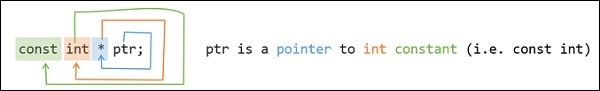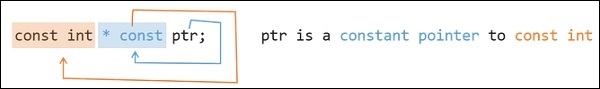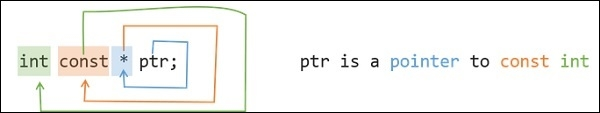# What is the difference between const int*, const int * const, and int const *?

Here we will see some different types of variable declaration based on integer pointers integer constants and the integer constant pointers.

To determine them we will use the Clockwise/Spiral Rule. By discussing the terms, we can understand the rules also.

The const int *. This is used to tell the compiler that this is a pointer type variable, and this can store address of some constant int. The Clock rule is saying like this −Now the another one is const int * const. This is used to denote that this is one constant pointer variable, which can store the address of another constant integer. The clock rule can be applied, and we can get the statement like below −The third one is int const*. This is used to denote the variable is a pointer type variable, and this can point to one constant int. The clock rule can be applied like below.If you notice, you can understand that the first one and the last one, (const int * and the int const *) are same. So we can use them interchangeably.